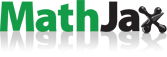# Doubly Recursive Integer Factorizations

As you know, every integer greater than $$1$$ can be uniquely factored into a product of powers of prime numbers: $$24 = 8 \times 3 = 2^3 \times 3^1$$, that kind of thing. No news there. Knowing that each prime number can be indexed, as the first prime $$p_1 = 2$$, the second prime $$p_2 = 3$$, the third prime $$p_3 = 5$$, the fourth prime $$p_4 = 7$$, and so on, each integer greater than $$1$$ can then be expressed as

$$\prod p_i^k$$

where $$i$$ is the index of the prime and $$k$$ is the exponent of the prime in the integer factorization. For example, $$24 = 8 \times 3 = 2^3 \times 3^1 = p_1^3 p_2^1$$. No news there either.

What you might not have tried before is to then do the same thing to the indexes and exponents: $$24 = 8 \times 3 = 2^3 \times 3^1 = p_1^3 p_2^1 = p_1^{p_2^1} p_{p_1^1}^1$$.

If you keep going, eventually all of the indexes and exponents will be $$1$$’s: $$24 = 8 \times 3 = 2^3 \times 3^1 = p_1^3 p_2^1 = p_1^{p_2^1} p_{p_1^1}^1 = p_1^{p_{p_1^1}^1} p_{p_1^1}^1$$.

Once you get that far, you might as well remove all of the 1’s because they don’t add any information, leaving you with $$p_1^{p_{p_1^1}^1} p_{p_1^1}^1 = p^{p_p} p_p$$. Using this procedure, any integer greater than 1 can be expressed using this kind of expression.

 $$2$$ $$= p_1 = p$$ $$3$$ $$= p_2 = p_p$$ $$4$$ $$= p_1^2 = p_1^{p_1} = p^p$$ $$5$$ $$= p_3 = p_{p_2} = p_{p_p}$$ $$6$$ $$= p_1 p_2 = p p_p$$ $$7$$ $$= p_4 = p_{p^p}$$ $$8$$ $$= p_1^3 = p^{p_2} = p^{p_p}$$ $$9$$ $$= p_2^2 = p_p^p$$ $$10$$ $$= p_1 p_3 = p p_{p_p}$$ $$11$$ $$= p_5 = p_{p_{p_p}}$$

Focusing on the shape of the expressions, towers of exponents look like this (just towers of powers of 2):

 $$p$$ $$= 2$$ $$p^p$$ $$= 2^2 = 4$$ $$p^{p^p}$$ $$= 2^{2^2} = 16$$ $$p^{p^{p^p}}$$ $$= 2^{2^{2^2}} = 65,536$$ $$p^{p^{p^{p^p}}}$$ $$= 2^{2^{2^{2^2}}} \approx 2.00352993 \times 10^{19,728}$$

And towers of indexes/subscripts look like this:

 $$p$$ $$= 2$$ $$p_p$$ $$= 3$$ $$p_{p_p}$$ $$= 5$$ $$p_{p_{p_p}}$$ $$= 11$$ $$p_{p_{p_{p_p}}}$$ $$= 31$$ $$p_{p_{p_{p_{p_p}}}}$$ $$= 127$$ $$p_{p_{p_{p_{p_{p_p}}}}}$$ $$= 709$$ $$p_{p_{p_{p_{p_{p_{p_p}}}}}}$$ $$= 5,381$$ $$p_{p_{p_{p_{p_{p_{p_{p_p}}}}}}}$$ $$= 52,711$$ $$p_{p_{p_{p_{p_{p_{p_{p_{p_p}}}}}}}}$$ $$= 648,391$$ $$p_{p_{p_{p_{p_{p_{p_{p_{p_{p_p}}}}}}}}}$$ $$= 9,737,333$$

This is OEIS sequence A007097, $$a(n+1)$$ is the $$a(n)$$th prime number.

Combined indexes and exponents:

 $$p_p^p$$ $$= p_2^2 = 3^2 = 9$$ $$p_{p_p}^{p^p}$$ $$= p_3^{2^2} = 5^4 = 625$$ $$p_{p_{p_p}}^{p^{p^p}}$$ $$= \ldots$$

Some of these expressions look like typeset versions of the Sierpinski gasket:

 $$p$$ $$= 2$$ $$p_p^p$$ $$= 9$$ $$p_{p_p^p}^{p_p^p}$$ $$= 1,801,152,661,463$$ $$p_{p_{p_p^p}^{p_p^p}}^{p_{p_p^p}^{p_p^p}}$$ $$= {55,125,235,480,573}^{1,801,152,661,463} \approx 10^{10^{13.39\ldots}}$$

Things might have got a little bit out of hand.

I didn’t really add anything to the work of J. Awbrey, Riffs and Rotes, but still.

Designed and rendered using Wolfram Mathematica 11, with first timer’s thanks to:.
First draft August 2018 by Robert Dickau.

[ home ] || [ 2018-08-14 ]

www.robertdickau.com/drif.html